# Robin constant

(diff) ← Older revision | Latest revision (diff) | Newer revision → (diff)

A numerical characteristic of a set of points in a Euclidean space,, closely connected with the capacity of the set.

Letbe a compact set in, and letbe a positive Borel measure concentrated onand normalized by the condition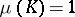. The integral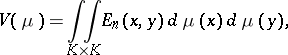where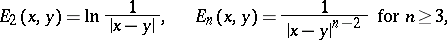and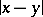is the distance between two points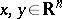, is the energy of(cf. Energy of measures). The Robin constant of the compact setis the lower boundover all measuresof the indicate type. If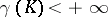, then this bound is finite and is attained for some (unique) equilibrium, or capacitary, measure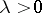,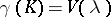,, concentrated on; if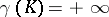, thenfor all measuresof the indicated type. The Robin constant ofis related to its capacity by the formula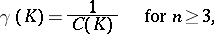If the boundaryofis sufficiently smooth, for example, if it consists of a finite number of pairwise non-intersecting simple closed surfaces (for) or curves (for) of class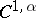,, then the equilibrium measureis concentrated on the partwhich forms the boundary of that connected component of the complement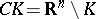which contains the point at infinity. The equilibrium potential, Robin potential or capacity potential, i.e. the potential of the equilibrium measure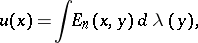in this case assumes a constant value on, equal to, which allows one to calculate the Robin constant of a compact set in the simplest cases (see Robin problem). For instance, the Robin constant of a disc of radius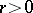in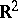is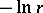, and the Robin constant of a ball of radiusin,, is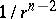. In the case of an arbitrary compact setof positive capacity,everywhere and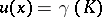everywhere on the support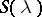of the equilibrium measure, except possibly at the points of some polar set; moreover,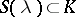.

Letbe a domain in the extended complex planecontaining inside it the point at infinity and having a Green function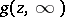with pole at infinity. Then the following representation holds:(1)

where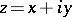is a complex variable,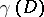is the Robin constant of the domainand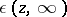is a harmonic function in; moreover,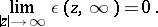The Robin constant of the domain, defined by (1), coincides with the Robin constant of the compact set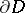: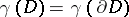. If the Green function for the domaindoes not exist, then one assumes that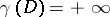.

By generalizing the representation (1) to a Riemann surfacewhich has a Green function, one can obtain a local representation of the Green function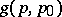with pole: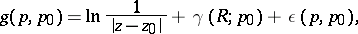(2)

where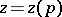is a local uniformizing parameter in a neighbourhood of the pole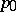,,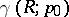is the Robin constant of the Riemann surfacerelative to the pole, and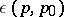is a harmonic function in a neighbourhood of; moreover,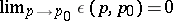. For Riemann surfaceswhich do not have a Green function one assumes. In expression (2) the value of the Robin constantdepends now on the choice of the pole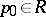. However, the relations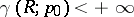and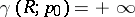are independent of the choice of the pole. This allows one to use the notion of a Robin constant in the classification of Riemann surfaces (cf. Riemann surfaces, classification of).

How to Cite This Entry:
Robin constant. Encyclopedia of Mathematics. URL: http://encyclopediaofmath.org/index.php?title=Robin_constant&oldid=18330
This article was adapted from an original article by E.D. Solomentsev (originator), which appeared in Encyclopedia of Mathematics - ISBN 1402006098. See original article